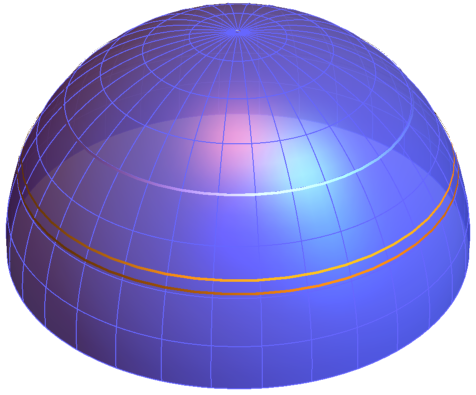While toying around with some bits-n-bobs for my next quaternion quantization post I made a joke twitter post:

Pointless 3D fact of the day: Given uniform random rotations, then their average is $\pi/2$ + $2/\pi$ radians (~126.476 degrees)

I was probably type parts of this up anyway, so I’ll just do it now…sorry it’s kinda brain-dump like.

## Prelim

Rehashing the same old stuff. The set of all quaternions $\mathbb{H}$, excluding zero, can represent 3D rotations by the similarity transform. The subset of quaternions representing the same rotation form a line through the origin (excluded). Limiting ourselves to unit quaternions this line intersects the 4D sphere (3-sphere or $\mathbb{S}^3$) at two points $Q$ and $-Q$. By further limiting ourselves to unit quaternions with positive (or zero) scalars then each point in this subset represents a unique 3D rotation. So unit quaternions lie on $\mathbb{S}^3$ and we can represent all rotations by half of this sphere. By a web-search or hitting up a math reference site we can finding the surface volume (or 3-dimensional cubic hyperarea if that’s your thing) of the sphere which is $2\pi^2$, so the volume of our half sphere is $\pi^2$. Gotta run through the math of this since we need the volume element.

## Attempt at human readable summary

I’m going to try summarize this post for a wide target audience. Let’s say we wanted to find the average angle of a uniform random rotation in two dimensions. This is the same as points uniformly distributed on the surface (arc lengths) of the unit circle and if we measure angles as minimum magnitude then the answer is the probably unsurprising value of $\frac{\pi}{2}$ radians. In three dimensions this is the same problem as uniform random rotations about a fixed axis. If we extend this to allow any axis of rotation bound to some plane (say the $\lbrace xy\rbrace$-plane) then the result is the same. This is equivalent to points uniformly distributed on the surface of the unit 3D sphere, choose a reference direction (logical choice is $+\mathbf{z}$) and finding the rotation that maps that direction $\left(\mathbf{z}\right)$ to the random point. I’ve skimmed the math of random generation a previous post Uniform points on disc, circle, sphere and caps. Additionally the median of both of these is $\frac{\pi}{2}$ as well. To be clear on terminology the average is: “let’s generate $n$ values and average their result and let $n$ go to infinity” and median is “let’s generate $n$ results, sort them and find the value at $\frac{n}{2}$”. So in terms of uniform distributed point-sets the median is the value (here angle) where the length/area/volume is divided in two.

Moving to the general case of 3D uniform random rotations is equivalent to points uniformly distributed on the 4D unit hemisphere. This is hard to visualize so let’s look at a 3D slice of it:The vertical axis is line of reals (aka scalars, typically called ‘w’) and the “north pole” is the quaternion value of one which is the identity (no rotation) transform. The other two dimensions can be any planar slice of what we’d typically think of as normal 3D space, but let’s say that it’s the $\lbrace xy\rbrace$-plane. This choice means that hemisphere we’re visualizing is the set of all rotations with an axis in the $\lbrace xy\rbrace$-plane, so the second example from above. This specialized case actually is a 3D hemisphere (the $z$ component of the quaternion is always zero) and the “white-ish” circle indicates the $\frac{\pi}{2}$ split which is at an angle of $\frac{\pi}{4}$ with respect to the real (vertical) axis because of the half/double angle relationship between 3D rotation angle and quaternion space measurements.

## Volume of $SO\left(3\right)$

Define a unit quaternion with the bivector part in azimuth $\left(\alpha\right)$ / inclination $\left(\beta\right)$ spherical coordinates:

with angle ranges of:

Now we have this 3D spherical coordinate thing that’s be converted into a 4D Euclidean space thing and I want to compute the Jacobian determinant. To get a square Jacobian (so we can compute the determinate) I’ll just extend the quaternion to 4D by allowing non-unit magnitude (or a radius if you want) and multply the components by $r$:

Then we have the Jacobian:

Setting $r=1$ then the volume element is:

The volume is then:

Great it agrees with what we could have looked up on Wikipedia (EDIT: MathWorld has the volume element)

## Volume of $SO\left(3\right)$ take 2

I don’t need it but since I’m typing we could have done this a second way. (Wikipedia, Encyclopedia of Mathematics) Without adding an extra dimension the Jacobian is:

then the metric tensor:

and it’s determinate:

The volume element is then:

Same as before.

## Average rotation angle of uniform random rotations

Not explictly mentioned so far is that $\theta$ is the measure in quaternion space which translates into a $2\theta$ rotation. I want to find the average rotation angle $\phi$ of a uniform distribution of random rotations. We have $q_w = \cos\left(\frac{\phi}{2}\right) = \cos\left(\theta\right)$ and the expected value is:

There’s surely an easier way to do this and I’m shaky on the math here, so an empirical validation: CLICK

EDIT: Same result discussed in this math.stackexchange post based on this paper (PDF). Also I was using probablity verbage here where I should have used geometric. I’m finding the “center-of-mass” of the point set since the “average” is summing up the angles and dividing by number of points (extended from discrete to continous).

## Cumulative volume of angle range volume of cap & median random rotation

Finding the volume of all 3D rotations with angle $\phi$ and less is simply changing the upper bound of the $\theta$ integral:

Solving the above for half the total volume of rotations gives $\phi \approx 2.30988$ radians (~132.35 degrees) which is the median angle of uniform random rotations.